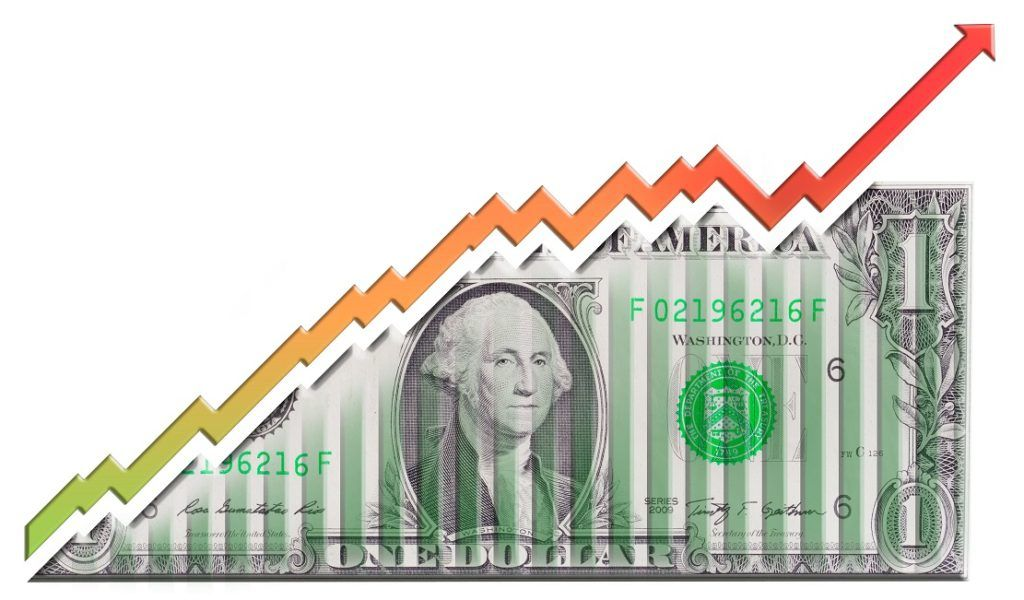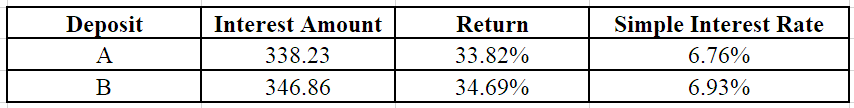# Continuously Compounded Interest

Interest that is computed on the initial principal as well as all interest earned by the principal in the past

## What is Continuously Compounded Interest?

Continuously compounded interest is interest that is computed on the initial principal, as well as all interest other interest earned. The idea is that a principle will receive interest at all points in time, rather than in a discrete way at certain points in time.The continuous payment of interest leads to exponential growth and is many times used as an argument for wealth creation. Albert Einstein is credited with the phrase “compound interest to be “the most powerful force in the universe,” while it is undetermined if he actually said it, it says a lot about the importance of the concept.

To understand continuously compounded interest, we will quickly review simple interest and compound interest.

Consider the following example: An investor invests \$1,000 in a 5-year term deposit that pays a continuously compounded interest of 6%.

### What is Simple Interest?

Simple interest is only computed on the initial principal and not on any interest earned by the initial principal amount. Consider the following example: An investor invests \$1,000 in a 5-year term deposit paying a simple interest of 6%.

### What is Compound Interest?

Compound interest is computed on the initial principal as well as on the interest earned by the principal over a specified period of time. Consider the following example: An investor invests \$1,000 in a 5-year term deposit with an interest rate of 8% with the interest compounded annually.

Therefore, at the end of each year, the interest amount generated in that year is added to the principal amount. It is the new principal amount and the interest for the next year is generated based on the principal amount.

### Formula for Compounded Interest

General compound interest takes into account interest earned over some previous interval of time.

##### General Compound Interest = Principal * [(1 + Annual Interest Rate/N)N*Time

Where:

• N is the number of times interest is compounded in a year.

Consider the following example: An investor is given the option of investing \$1,000 for 5 years in two deposit options.

• Deposit A pays 6% interest with the interest compounded annually.
• Deposit B pays 6% interest with the interest compounded quarterly.Clearly, Deposit B is a better option as it provides a higher return.

### Continuously Compounded Interest Formula

Continuously compounded interest is the mathematical limit of the general compound interest formula with the interest compounded an infinitely many times each year. Or in other words you are paid every possible time increment. Mathematicians, have derived a way to approximate the value such a sum would converge to, and it is given by the following formula:Where:

• N is the number of times interest is compounded in a year.

Continuously compounded interest is the mathematical limit of the general compound interest formula with the interest compounded an infinitely many times each year. Consider the example described below.

• Initial principal amount is \$1,000.
• Rate of interest is 6%.
• The deposit is for 5 years.

### Table of Interest Payments and Total Return

Consider the example described above.

• Initial principal amount is \$1,000.
• Rate of interest is 6%.
• The deposit is for 5 years.

No. of Compounding Periods Each YearInterest AmountReturn (in %)
1338.2256
33.82256
2343.916434.39164
3345.868334.58683
4346.85534.6855
5347.450534.74505
6347.848934.78489
7348.134234.81342
8348.348634.83486
9348.515634.85156
10348.649334.86493
11348.758834.87588
12348.850234.88502
13348.927534.89275
14348.993834.89938
15349.051334.90513
16349.101634.91016
17349.14634.9146
18349.185534.91855
19349.220934.92209
20349.252734.92527
100349.737434.97374
1,000349.846734.98467
10,000349.857634.98576
100,000349.858734.98587

### More Resources

CFI is the official provider of the Financial Modeling and Valuation Analyst (FMVA)™ certification program, designed to transform anyone into a world-class financial analyst.

To keep learning and developing your knowledge of financial analysis, we highly recommend the additional resources below:

• Math for Corporate Finance Course
• Continuously Compounded Return
• Effective Annual Interest Rate
• Interest Income

### Financial Analyst Training

Get world-class financial training with CFI’s online certified financial analyst training program!

Gain the confidence you need to move up the ladder in a high powered corporate finance career path.

Learn financial modeling and valuation in Excel the easy way, with step-by-step training.